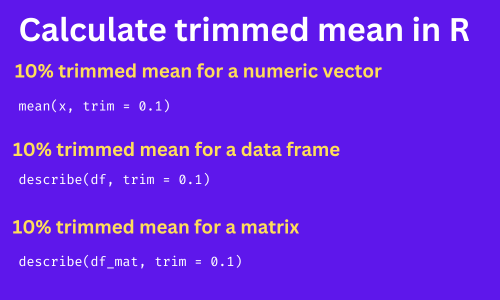# How to Compute Trimmed Mean in R?Trimmed mean is an arithmetic mean which is calculated by removing the specific percentages of the largest and smallest values from the sample. The trimmed mean is also known as the truncated mean.

A 10% trimmed mean is calculated using the remaining 80% of data after removing 10% of the largest and smallest values.

Trimmed mean is a robust measure of central tendency and removes the effect of erratic observations such as outliers.

The trimmed mean could be calculated by removing the 5%, 10%, 20%, etc. largest and smallest values from the sample.

In R, trimmed mean can be calculated for vector, data frame, and matrix using various functions such as `mean()` and `describe()`.

The syntax for the `mean()` function to calculate the trimmed mean is,

``````# calculate 5% trimmed mean
mean(x, trim = 0.05)

# calculate 10% trimmed mean
mean(x, trim = 0.1)
``````

In the above function, `x` is an object which could be a numeric vector or data frame column. The `trim` parameter is used for calculating certain percentages of the trimmed mean.

If `trim = 0` (default value), the `mean()` function returns the arithmetic mean of the `x`.

The following examples illustrate how to calculate the trimmed mean using `mean()` and `describe()` functions.

## 1. Compute trimmed mean for a vector

The following example shows how to calculate the 5% trimmed mean on a numeric vector. If you have a character or alphanumeric vector, it will return `NA`.

``````# create a random numeric vector
x <- c(1, 2, 4, 10, 4, 5, 0.5, 8)

# calculate 5% trimmed mean
mean(x, trim = 0.05)
4.3125
``````

The 5% trimmed mean is 4.3125 after removing 5% largest and smallest values from the vector.

In addition, to the `mean()` function, you can also use `describe()` function (from the `psych` package) to calculate the trimmed mean of a vector. By default, it calculates the 10% trimmed mean.

``````# create a random numeric vector
x <- c(1, 2, 4, 10, 4, 5, 0.5, 8)

library(psych)

# calculate 10% trimmed mean
describe(x)

vars n mean   sd median trimmed  mad min max range skew kurtosis   se
X1    1 8 4.31 3.33      4    4.31 3.71 0.5  10   9.5 0.44    -1.38 1.18

# calculate 20% trimmed mean
describe(x, trim = 0.2)

vars n mean   sd median trimmed  mad min max range skew kurtosis   se
X1    1 8 4.31 3.33      4       4 3.71 0.5  10   9.5 0.44    -1.38 1.18
``````

The 10% trimmed mean is 4.31 and the 20% trimmed mean is 3.71.

## 2. Calculate the trimmed mean for a data frame

The following example shows how to calculate the trimmed mean for specific columns or all columns of a data frame.

``````# load example dataset

# view data frame
df
A  B  C  D
1 25 45 30 54
2 30 55 29 60
3 28 29 33 51
4 36 56 37 62
5 29 40 27 73

# calculate 10% trimmed mean for column A
mean(df\$A, trim = 0.1)
29.6
``````

The 10% trimmed mean for an `A` column is 29.6.

You could also use `describe()` function to get the trimmed mean for all columns at once. For example, calculate the 10% trimmed mean for all columns for a following example data frame

``````# load example dataset

# view data frame
df
A  B  C  D
1 25 45 30 54
2 30 55 29 60
3 28 29 33 51
4 36 56 37 62
5 29 40 27 73

library(psych)

# calculate 10% trimmed mean for all columns
describe(df)

vars n mean    sd median trimmed   mad min max range  skew kurtosis   se
A    1 5 29.6  4.04     29    29.6  1.48  25  36    11  0.49    -1.39 1.81
B    2 5 45.0 11.20     45    45.0 14.83  29  56    27 -0.27    -1.85 5.01
C    3 5 31.2  3.90     30    31.2  4.45  27  37    10  0.39    -1.72 1.74
D    4 5 60.0  8.51     60    60.0  8.90  51  73    22  0.41    -1.61 3.81
``````

The trimmed column shows the 10% trimmed mean for all columns in a data frame.

## 3. Calculate the trimmed mean for a matrix

The `describe()` function could be used for calculating the trimmed mean on matrix.

The following examples show how to calculate the trimmed mean on the matrix,

``````# load package
library(psych)

# convert to matrix
df_mat = data.matrix(df)

# get summary statistics
describe(df_mat)

vars n mean    sd median trimmed   mad min max range  skew kurtosis   se
A    1 5 29.6  4.04     29    29.6  1.48  25  36    11  0.49    -1.39 1.81
B    2 5 45.0 11.20     45    45.0 14.83  29  56    27 -0.27    -1.85 5.01
C    3 5 31.2  3.90     30    31.2  4.45  27  37    10  0.39    -1.72 1.74
D    4 5 60.0  8.51     60    60.0  8.90  51  73    22  0.41    -1.61 3.81
``````

The trimmed column shows the 10% trimmed mean for all columns in a matrix.